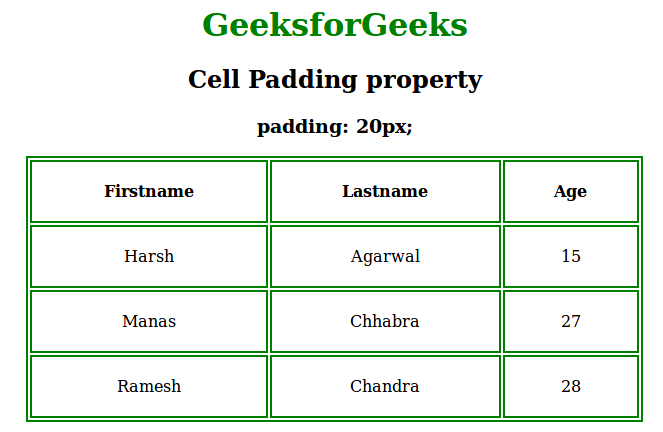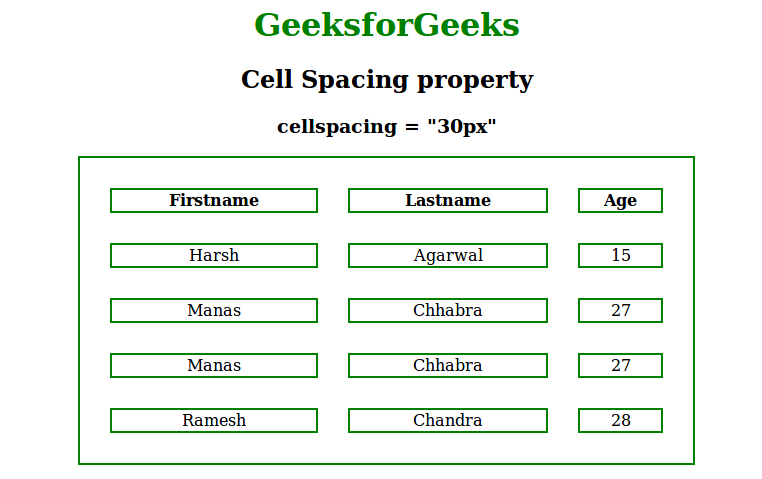# Set cellpadding and cellspacing in CSS

The cell padding is used to define the spaces between the cells and its border. If cell padding property is not applied then it will be set as the default value.

Example:

## html

 `` `<``html``>`   `<``head``>` `    ``<``title``>cell padding` `    ``<``style``>` `        ``table, th, td {` `            ``border: 2px solid green;` `            ``text-align:center;` `        ``}` `        ``th, td {` `            ``padding: 20px;` `            ``background-color:none;` `        ``}` `        `  `        ``h1 {` `        ``color:green;` `        ``}` `    ``` ``   `<``body``>` `    ``<``center``>` `        ``<``h1``>GeeksforGeeks` `        ``<``h2``>Cell Padding property` `        ``<``h3``>padding: 20px;` `        ``<``table` `style``=``"width:70%"``>` `            ``<``tr``>` `                ``<``th``>Firstname` `                ``<``th``>Lastname` `                ``<``th``>Age` `            ``` `            ``<``tr``>` `                ``<``td``>Harsh` `                ``<``td``>Agarwal` `                ``<``td``>15` `            ``` `            ``<``tr``>` `                ``<``td``>Manas` `                ``<``td``>Chhabra` `                ``<``td``>27` `            ``` `            ``<``tr``>` `                ``<``td``>Ramesh` `                ``<``td``>Chandra` `                ``<``td``>28` `            ``` `        ``` `    ``` ``   ``

Output:Cell Spacing

The cell spacing is used to define the space between the cells.

Example:

## html

 `` `<``html``>`   `<``head``>` `    ``<``title``>cell spacing property` `    ``<``style``>` `        ``table, th, td {` `            ``border: 2px solid green;` `            ``text-align:center;` `        ``}` `        ``h1 {` `        ``color:green;` `        ``}` `    ``` ``   `<``body``>` `    ``<``center``>` `        ``<``h1``>GeeksforGeeks` `        ``<``h2``>Cell Spacing property` `        ``<``h3``>cellspacing = "30px"` `        ``<``table` `style``=``"width:70%;"` `               ``cellspacing``=``"30px"``>` `            ``<``tr``>` `                ``<``th``>Firstname` `                ``<``th``>Lastname` `                ``<``th``>Age` `            ``` `            ``<``tr``>` `                ``<``td``>Harsh` `                ``<``td``>Agarwal` `                ``<``td``>15` `            ``` `            ``<``tr``>` `                ``<``td``>Manas` `                ``<``td``>Chhabra` `                ``<``td``>27` `            ``` `            ``<``tr``>` `                ``<``td``>Manas` `                ``<``td``>Chhabra` `                ``<``td``>27` `            ``` `            ``<``tr``>` `                ``<``td``>Ramesh` `                ``<``td``>Chandra` `                ``<``td``>28` `            ``` `        ``` `    ``` ``   ``

Output:Supported Browsers:

• Apple Safari